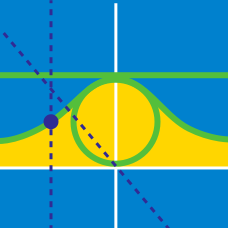Calculus

# Parametric Equations - Arc Length

The location of a dot $P$ at a given time $t$ in the $xy$ plane is given by $(x,y) = (t - \sin t, 1 - \cos t)$. What is the distance traveled by $P$ in the interval $0 \leq t \leq 2\pi$?

What is the length of the curve parametrized by the equations $\begin{array}{c}\displaystyle x=e^{2t}\cos t, & y=e^{2t}\sin t,\end{array}$ in the domain $0 \leq t \leq 4 ?$

If $x=4\sin^2 t$ and $y=4\cos^2 t,$ what is the distance traveled by the point $P=(x,y)$ during the time interval $0 \leq t \leq 5\pi?$

Given the curve $H(t) = \frac{2}{3} (t+4)^{3/2}$, the arc length of the graph between $t=4$ and $t=12$ can be expressed in the form $\frac {a\sqrt{b}}{c} - d$ where $a$, $b$, $c$, and $d$ are positive integers, $a$ and $c$ are coprime, and $b$ is not divisible by the square of any prime. What is $a+b+c+d$?

Given the curve defined by $x = t^3$ and $y = t^2,$ what is the length of the curve from $t=0$ to $t= 10?$

×